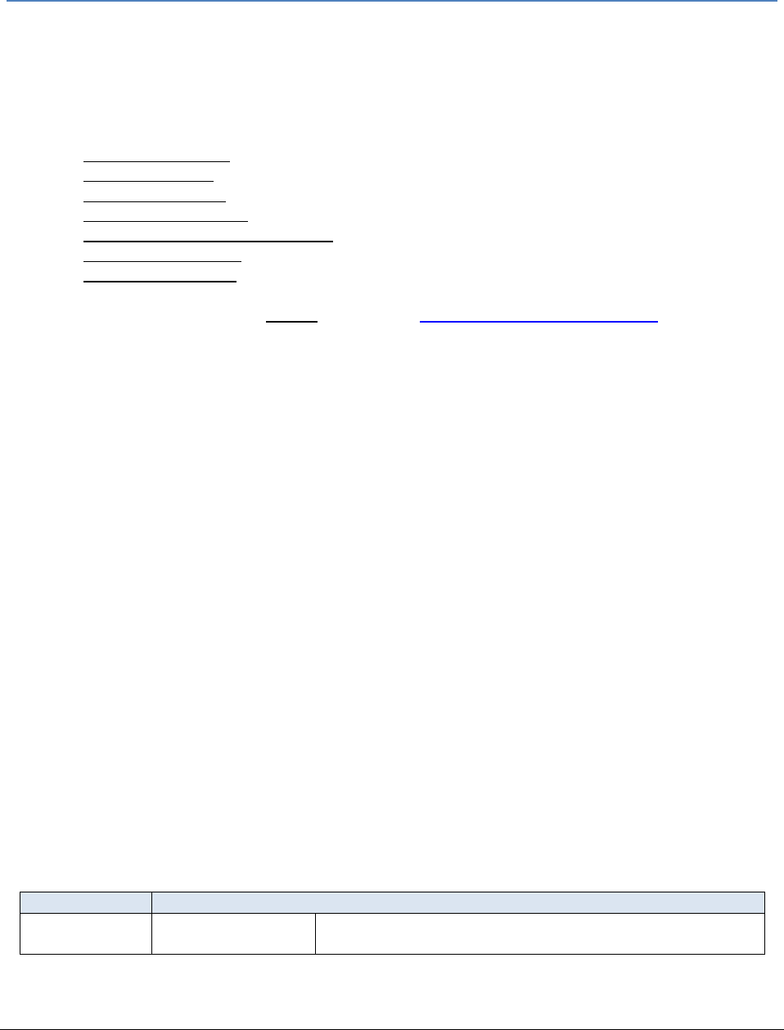# PHY 1122 Lecture Notes - Lecture 5: Calipers, Length Measurement, Graduated Cylinder

203 views5 pages
School
Department
CourseSimple measurements & buoyancy force
1
Simple measurements & buoyancy force
Introduction
The purpose of this experiment is to practice basic experimental measurements, calculation techniques and result
presentation methods (tables, graphs, etc.) that will be useful during the whole semester. Most of the subjects
covered during this experiment are presented in the following tutorials:
Measuring techniques
Experimental errors
Propagation of errors
Repeated measurements
How to present a calculation example
How to prepare a graph
How to prepare a table
These documents are available in the Tutorial section at the BbLearn physics laboratory website. Students should
read these tutorials before coming to their lab session.
Part 1 Length measurement
In this part, you will make length measurements using a meter stick and a vernier caliper. You will learn how to
report the uncertainty of your measurements and how to use them to perform error calculations when calculating
the density of some unknown materials.
Part 2 Time measurement
For this part, you will carry out several time measurements of the period of oscillation of a pendulum. You will use
these repeated measurements to calculate statistical quantities such as the average, the standard deviation and
the standard error.
Part 3 The buoyancy force
In this last part, you will investigate Archimedes’ principle which states that the buoyant force, 𝐹𝑏, exerted on an
object partially or fully submerged in a fluid will be equal to the weight of the fluid displaced by the object:
𝐹𝑏=𝜌𝑉𝑔 ,
(eq. 1)
where 𝜌 is the density of the fluid, 𝑉 is the volume of fluid displaced by the object, and 𝑔 is the gravitational
acceleration of 9.81 m/s2. You will use a suspended force sensor to measure the buoyancy force experienced by an
object that is gradually immersed in water. You will plot a graph of buoyancy force vs. the volume of displaced
liquid and, by using a linear regression tool, you will determine the value of the density of water, 𝜌.
Suggested reading
Students taking
Suggested reading
PHY 1122
Section 12.3
Unlock document

This preview shows pages 1-2 of the document.
Unlock all 5 pages and 3 million more documents.

Already have an account? Log inSimple measurements & buoyancy force
2
Objectives
Part 1 Length measurement
Familiarize yourself with different measuring instruments such as the meter stick and the vernier caliper.
Determine the uncertainty on each measurement and perform some error calculations based on length
measurements.
Prepare a proper table to report measurements.
Calculate the density of a metallic object and determine its metal types from a table.
Part 2 Time measurement
Calculate the period of oscillation of a pendulum.
Calculate the average, the standard deviation and the standard error of several measurements.
Learn how to describe and account for variation in a set of measurements.
Learn how to describe a range of experimental values.
Part 3 The buoyancy force
Determine the value of the density of a liquid using a force sensor to measure the buoyancy of an object
gradually being submerged in water.
Prepare a proper graph using Logger Pro and use the linear regression tool.
Compare your value with the accepted value for this quantity.
Materials
Meter stick and vernier caliper
Solid cylinder to measure the dimensions
Stopwatch
Pendulum
Electronic balance (one per classroom)
Computer equipped with Logger Pro and a Vernier computer interface
Force sensor
Lab jack with universal support and clamp holders
String and masses (2 x 200 g, 1 x 100 g)
Graduated cylinder
Safety warnings
Be careful not to drop the mass from the pendulum on your foot (you should always be wearing covered shoes in a
lab). Be careful to not spill water.
References for this manual
Density set. PASCO scientific (1992).
Unlock document

This preview shows pages 1-2 of the document.
Unlock all 5 pages and 3 million more documents.

Already have an account? Log in

# Get access

Grade+
\$10 USD/m
Billed \$120 USD annually
Homework Help
Class Notes
Textbook Notes
40 Verified Answers
Study Guides
1 Booster Class
Class+
\$8 USD/m
Billed \$96 USD annually
Homework Help
Class Notes
Textbook Notes
30 Verified Answers
Study Guides
1 Booster Class